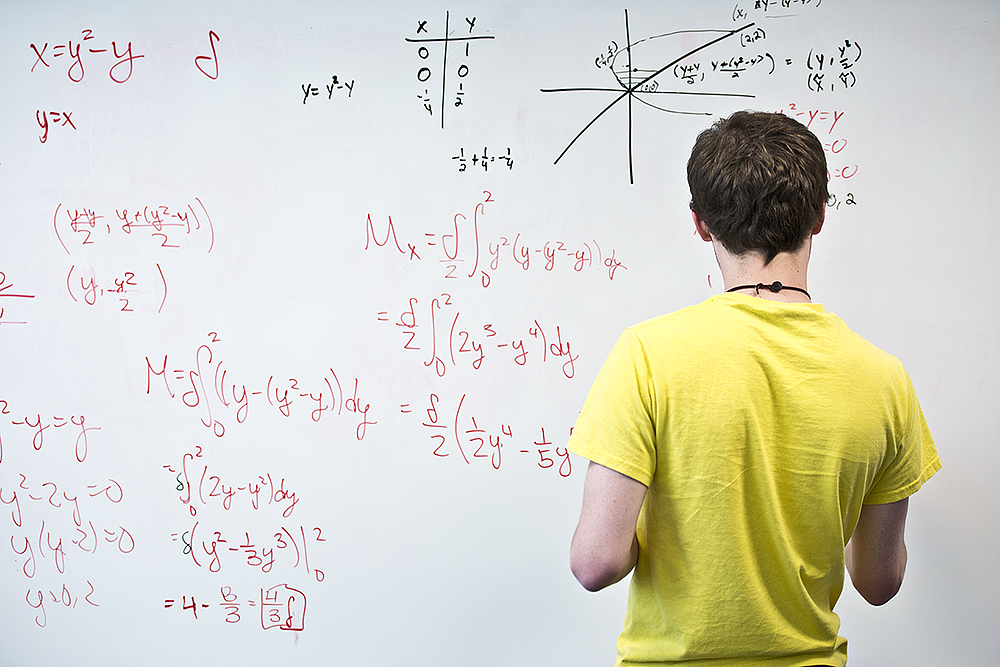# Here’s a list of math classes offered at KCC this summerRegistration for the Summer 2018 semester is open now! For information about signing up for summer classes, visit www.kellogg.edu/registration. To apply to KCC online for free, visit www.kellogg.edu/step1.

Interested in math? Here’s a list of several math (MATH) classes offered at Kellogg Community College during the Summer 2018 semester. For a complete list of Summer 2018 semester classes offered at KCC, visit www.kellogg.edu and click on “Class Schedules” in the top menu to search for sections.

#### MATH 102: Practical Algebra (4 Credits)

This course is designed for students pursuing a field of study outside of mathematics, science and engineering. Mathematical application will be an emphasis and real-life applications will be presented in disciplinary and career context when appropriate. Topics include: a review of operations with integers and rational numbers, ratios and proportions, percent, solutions of linear and quadratic equations, graphs of linear and exponential functions, linear and exponential models, introduction to data and measures of central tendency.

#### MATH 105: Beginning Algebra (4 Credits)

Course content includes operations on integers and rational numbers, geometric formulas, algebraic expressions, solutions of linear equations and inequalities, graphs of linear equations and linear systems, systems of linear equations in two variables, polynomials and factoring, rational expressions and equations, and radical expressions and equations.

#### MATH 125: College Algebra (4 Credits)

Topics included are subsets of the number system, relations and functions, linear systems of equations, polynomials, rational expressions and equations, exponents and radicals, complex numbers, polynomial equations, exponential and logarithmic functions and equations, applications, conic sections, matrices, binomial expansion, and families of functions of graphs.

#### MATH 130: Statistics (3 Credits)

A study of basic descriptive statistics, introduction to probability, probability distributions, sampling theory, hypothesis testing, analysis distributions, sampling theory, hypothesis testing, analysis of variance, and linear correlation and regression. Students are required to have a graphing calculator. Specifications will be made by the instructor.

#### MATH 132: Trigonometry (3 Credits)

This course is a study of trigonometric functions, their inverses and graphs, identities, equations, radian measure, and solution of triangles. Students are required to have a graphing calculator. Specifications will be made by the instructor.

#### MATH 140: Preparation for Calculus (4 Credits)

Topics in this course include: introductory plane geometry, algebraic functions and their graphs, introduction to theory of equations, combinations and binomial theorem, exponential and logarithmic functions, trigonometric functions, and arithmetic and geometric sequences. Students are required to have a graphing calculator. Specifications will be made by the instructor.

#### MATH 141: Calculus 1 (5 Credits)

Topics in this course include: limits, differentiation of algebraic and transcendental functions, the definite integral, fundamental theorem of calculus, and applications. Students are required to have a graphing calculator. Specifications will be made by the instructor.

#### MATH 142: Calculus 2 (5 Credits)

A study of the techniques of integration, limits, series, and applications. Students are required to have a graphing calculator. Specifications will be made by the instructor.

The first Summer 2018 classes start May 14, and the semester ends Aug. 8. For information about signing up for summer classes, visit www.kellogg.edu/registrationFor more information about studying math and science at KCC, visit www.kellogg.edu/math-science.